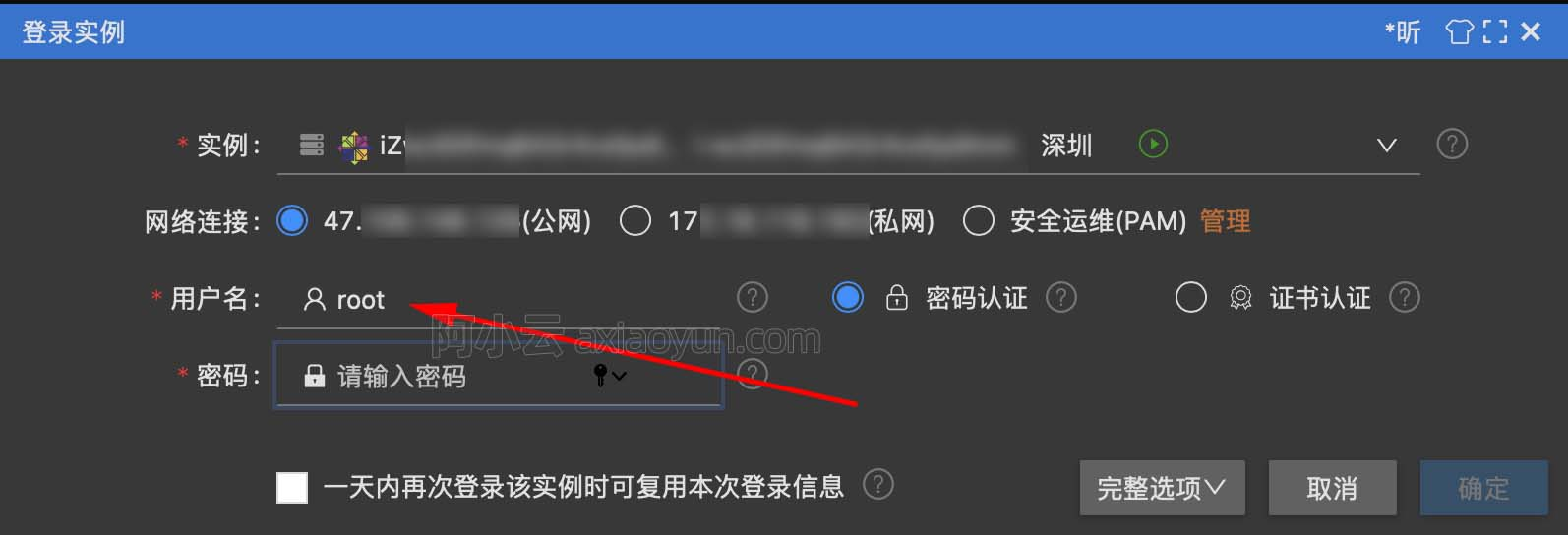# MMsegmentation教程 5: 训练技巧

+关注继续查看

MMSegmentation 支持如下训练技巧：

## 主干网络和解码头组件使用不同的学习率 (Learning Rate, LR)

optimizer=dict(
paramwise_cfg = dict(
custom_keys={
'head': dict(lr_mult=10.)}))

## 在线难样本挖掘 (Online Hard Example Mining, OHEM)

_base_ = './pspnet_r50-d8_512x1024_40k_cityscapes.py'
model=dict(
sampler=dict(type='OHEMPixelSampler', thresh=0.7, min_kept=100000)) )

## 类别平衡损失 (Class Balanced Loss)

_base_ = './pspnet_r50-d8_512x1024_40k_cityscapes.py'
model=dict(
loss_decode=dict(
type='CrossEntropyLoss', use_sigmoid=False, loss_weight=1.0,
# DeepLab 对 cityscapes 使用这种权重
class_weight=[0.8373, 0.9180, 0.8660, 1.0345, 1.0166, 0.9969, 0.9754,
1.0489, 0.8786, 1.0023, 0.9539, 0.9843, 1.1116, 0.9037,
1.0865, 1.0955, 1.0865, 1.1529, 1.0507])))

class_weight 将被作为 weight 参数，传递给 CrossEntropyLoss。详细信息请参照 PyTorch 文档

## 同时使用多种损失函数 (Multiple Losses)

_base_ = './fcn_unet_s5-d16_64x64_40k_drive.py'
model = dict(
dict(type='DiceLoss', loss_name='loss_dice', loss_weight=3.0)]),
dict(type='DiceLoss', loss_name='loss_dice', loss_weight=3.0)]),
)19825 064001 029215 022543 0Xshell使用SSH远程登录阿里云ECS服务器CentOS7
22365 016489 020725 015967 014905 023590 0
400

0

JS零基础入门教程（上册）